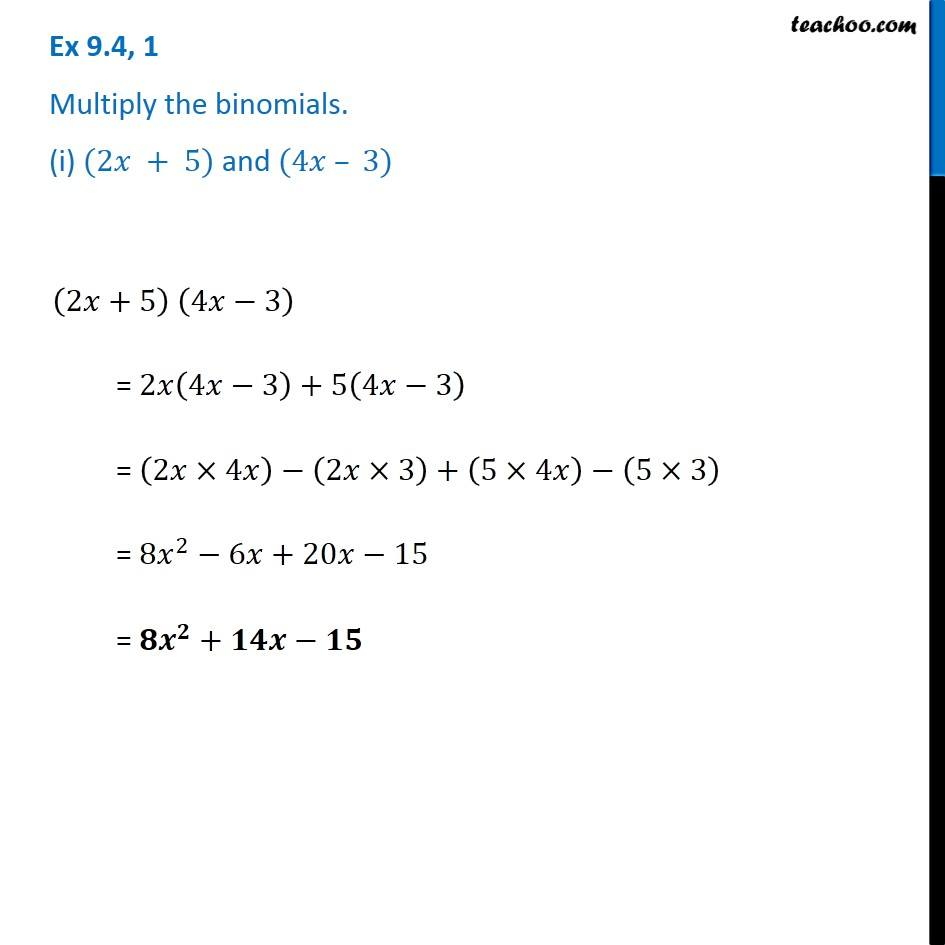1. Chapter 9 Class 8 Algebraic Expressions and Identities
2. Serial order wise
3. Ex 9.4

Transcript

Ex 9.4, 1 Multiply the binomials. (i) (2𝑥 + 5) and (4𝑥 – 3) (2𝑥+5) (4𝑥−3) = 2𝑥(4𝑥−3)+5(4𝑥−3) = (2𝑥×4𝑥)−(2𝑥×3)+(5×4𝑥)−(5×3) = 8𝑥^2−6𝑥+20𝑥−15 = 𝟖𝒙^𝟐+𝟏𝟒𝒙−𝟏𝟓

Ex 9.4